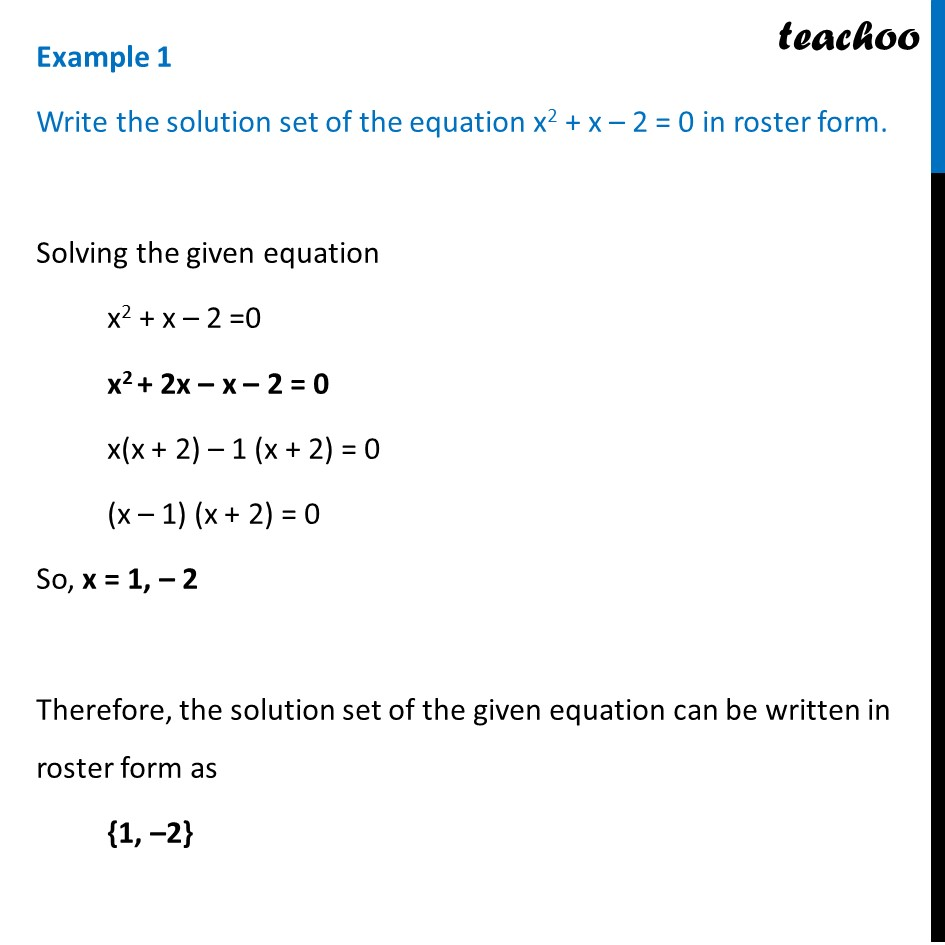Examples

Chapter 1 Class 11 Sets
Serial order wiseLearn in your speed, with individual attention - Teachoo Maths 1-on-1 Class

### Transcript

Example 1 Write the solution set of the equation x2 + x – 2 = 0 in roster form. Solving the given equation x2 + x – 2 =0 x2 + 2x – x – 2 = 0 x(x + 2) – 1 (x + 2) = 0 (x – 1) (x + 2) = 0 So, x = 1, – 2 Therefore, the solution set of the given equation can be written in roster form as {1, –2}